Home | ARTS | Operations Management | Problem of Critical Path Method (CPM)

# Problem of Critical Path Method (CPM)

Posted On :  23.06.2018 10:50 pm

Determine the critical path, the critical activities and the project completion time.

Problem 1

The following details are available regarding a project: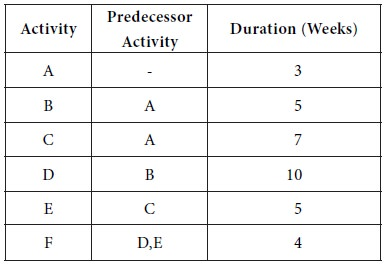Determine the critical path, the critical activities and the project completion time.

Solution

First let us construct the network diagram for the given project. We mark the time estimates along the arrows representing the activities. We obtain the following diagram: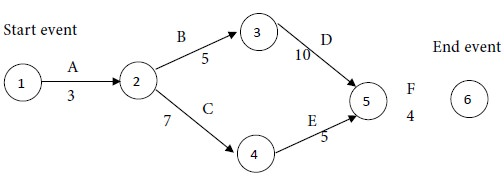Consider the paths, beginning with the start node and stopping with the end node. There are two such paths for the given project. They are as follows: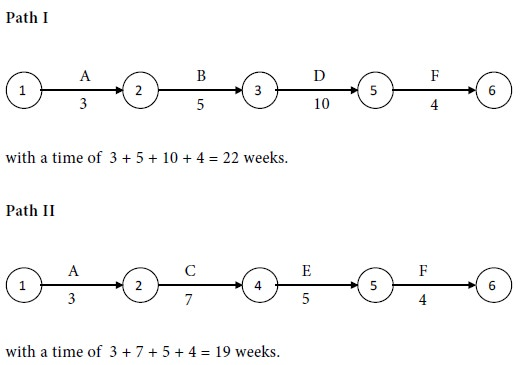Compare the times for the two paths. Maximum of {22,19} = 22. We see that path I has the maximum time of 22 weeks. Therefore, path I is the critical path. The critical activities are A, B, D and F. The project completion time is 22 weeks.

We notice that C and E are non- critical activities.

Time for path I - Time for path II = 22- 19 = 3 weeks.

Therefore, together the non- critical activities can be delayed upto a maximum of 3 weeks, without delaying the completion of the whole project.

Problem 2

Find out the completion time and the critical activities for the following project: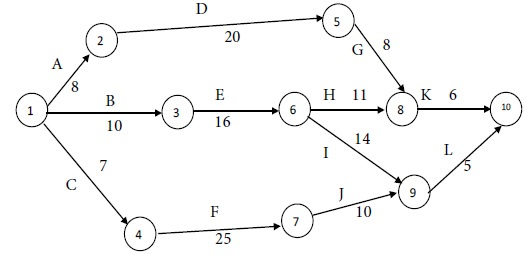Solution

In all, we identify 4 paths, beginning with the start node of 1 and terminating at the end node of 10. They are as follows: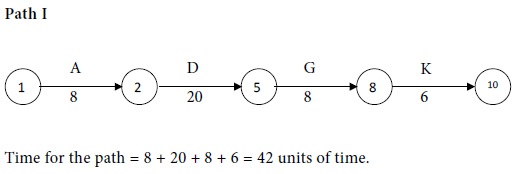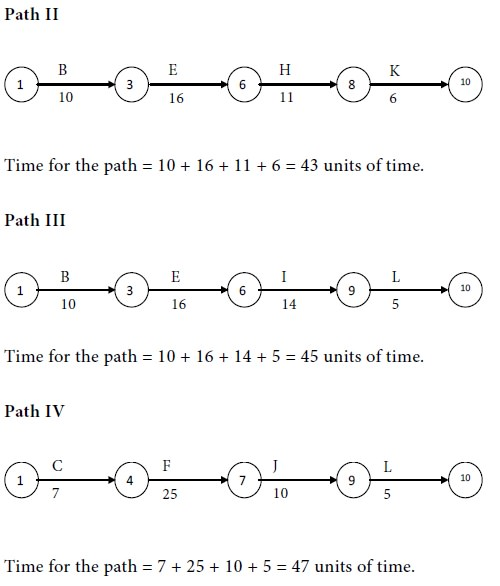Compare the times for the four paths. Maximum of {42, 43, 45, 47} = 47. We see that the following path has the maximum time and so it is the critical path: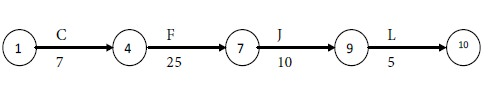The critical activities are C, F, J and L. The non-critical activities are A, B, D, E, G, H, I and K. The project completion time is 47 units of time.

Problem 3

Draw the network diagram and determine the critical path for the following project: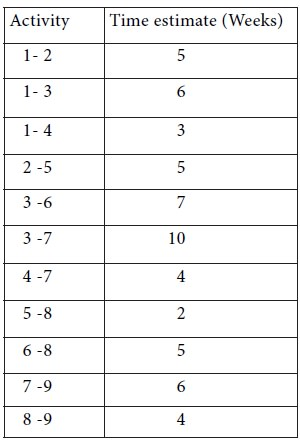Solution

We have the following network diagram for the project: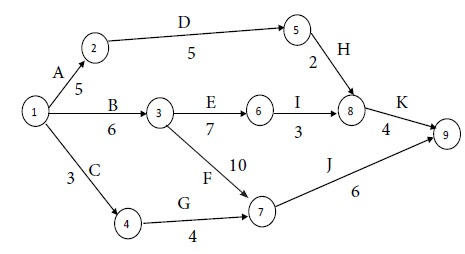Solution

We assert that there are 4 paths, beginning with the start node of 1 and terminating at the end node of 9. They are as follows: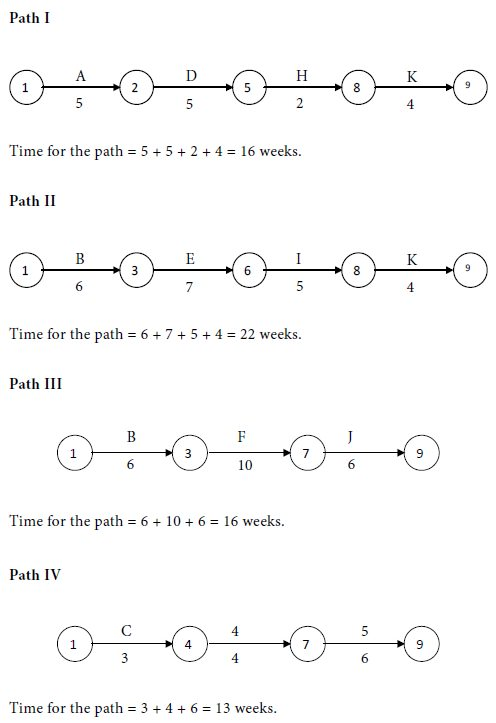Compare the times for the four paths. Maximum of {16, 22, 16, 13} =  22. We see that the following path has the maximum time and so it is the critical path: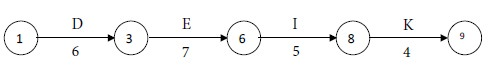The critical activities are B, E, I and K. The non-critical activities are A, C, D, F, G, H and J. The project completion time is 22 weeks.

Tags : Operations Management - Network Problems
Last 30 days 58020 views